Select Page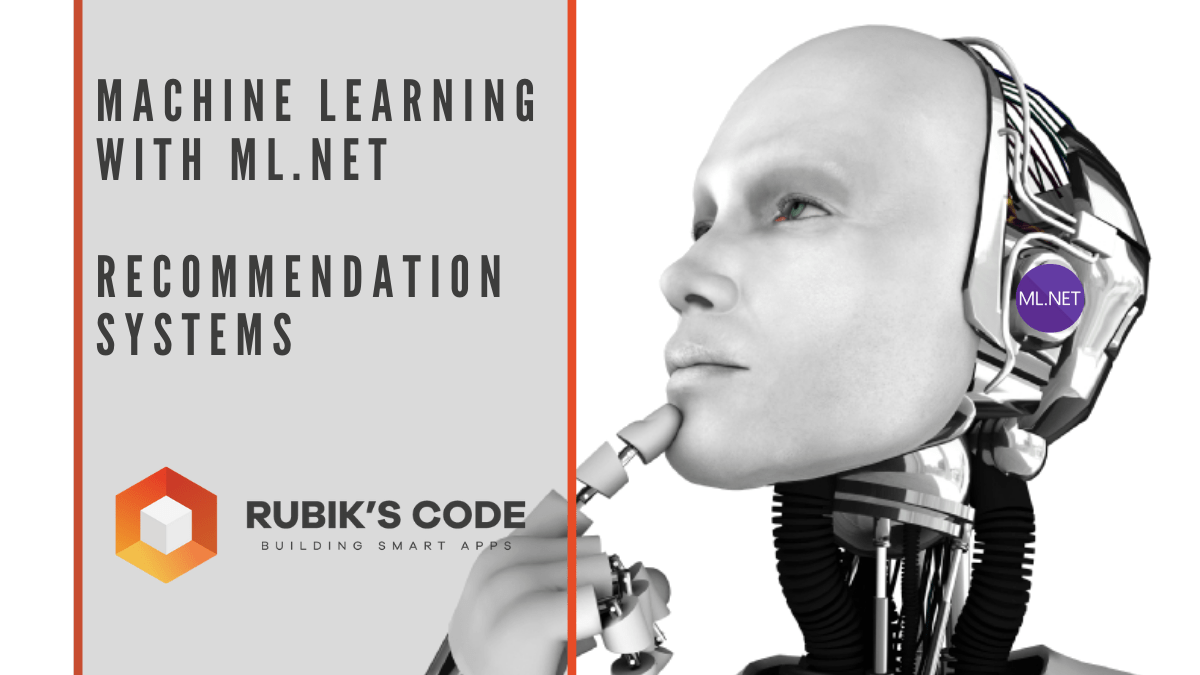## Machine Learning with ML.NET – Recommendation Systems

From Netflix, Google, and Amazon, to smaller webshops, recommendation systems are everywhere. In fact, this type of system represents probably one of the most successful business applications of Machine Learning. Their ability to predict what users would like to read,...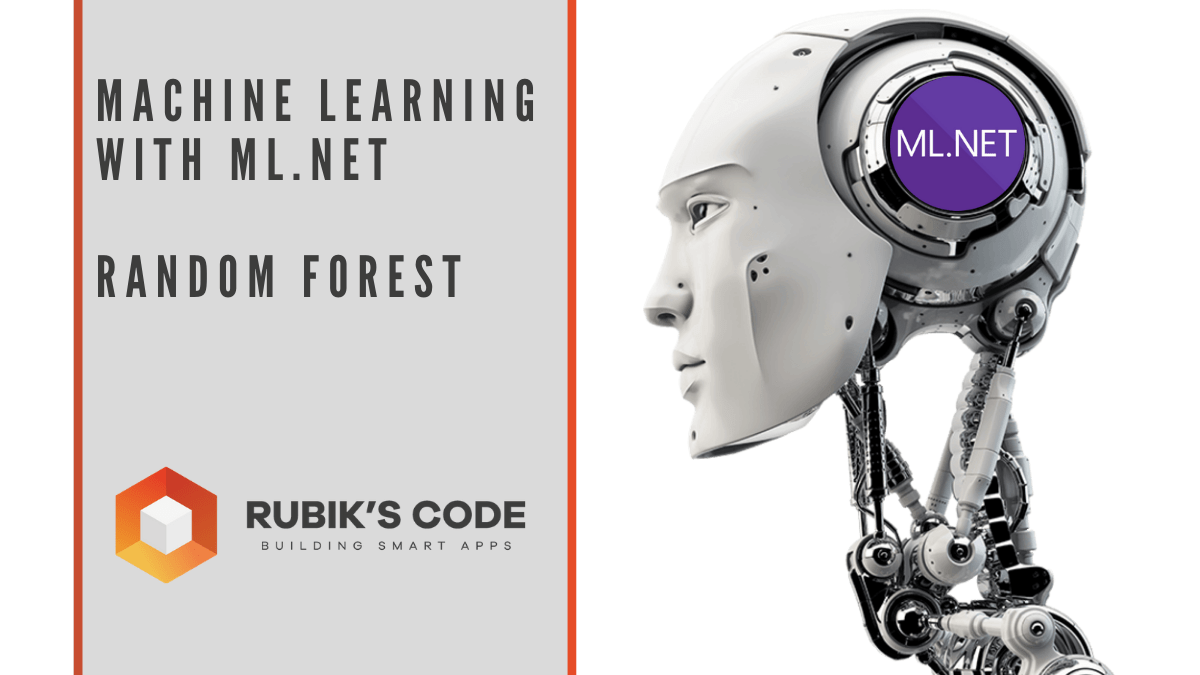## Machine Learning with ML.NET – Random Forest

In a previous couple of articles, we explored some basic machine learning algorithms and how they fit into the .NET world. Thus far we covered some simple regression algorithms, classification algorithms. Apart from that, we learned a bit about unsupervised learning,...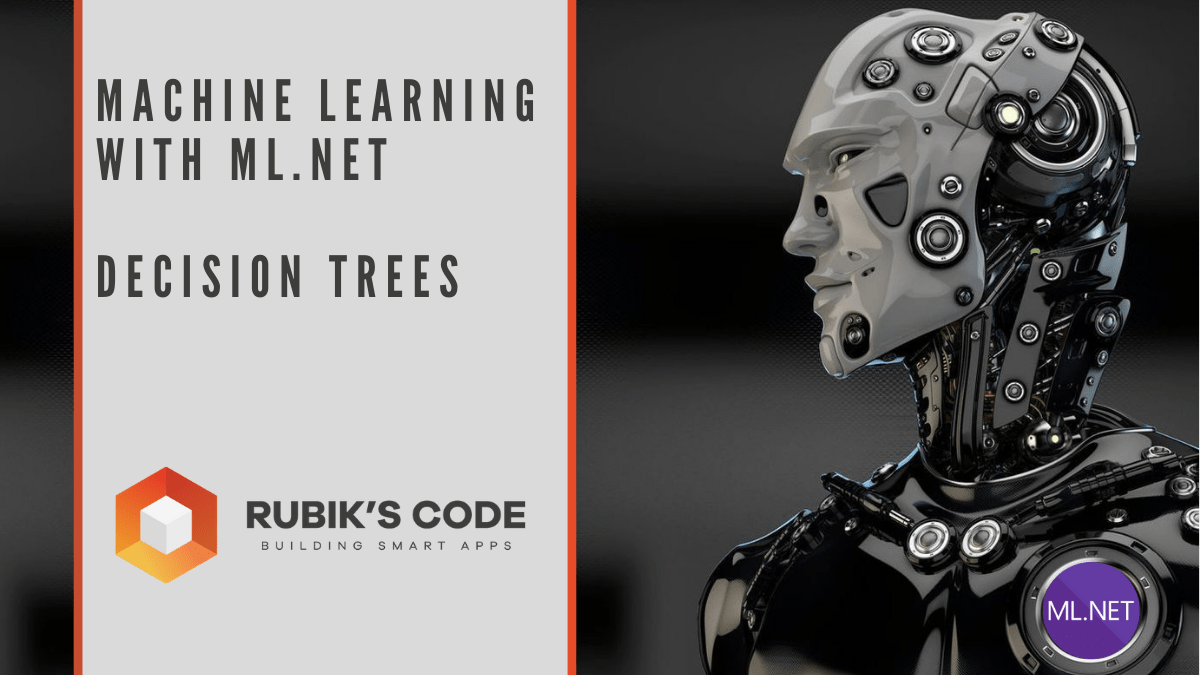## Machine Learning with ML.NET – Guide to Decision Trees

In a previous couple of articles, we explored some basic machine learning algorithms and how they fit into the .NET world. Thus far we covered some simple regression algorithms, classification algorithms. Apart from that, we learned a bit about unsupervised learning,...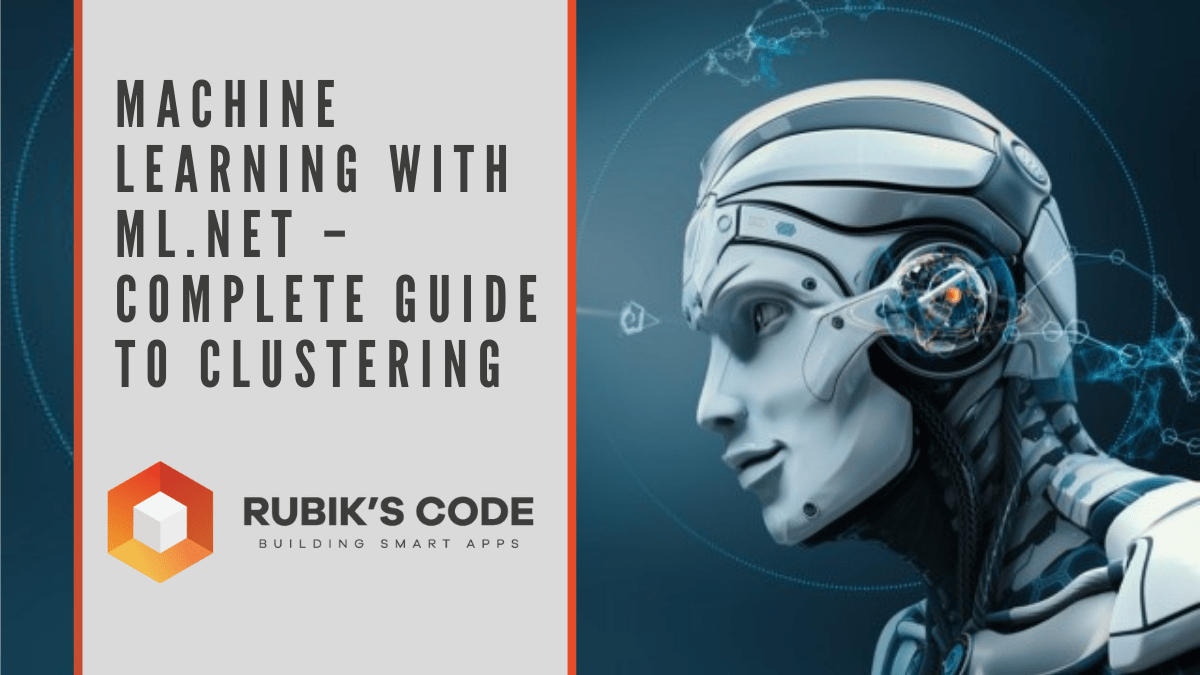## Machine Learning with ML.NET – Complete Guide to Clustering

In a previous couple of articles, we explored some basic machine learning algorithms. Thus far we covered some simple regression algorithms, classification algorithms. We used ML.NET implementation and application of these algorithms. Up to this point, we explored...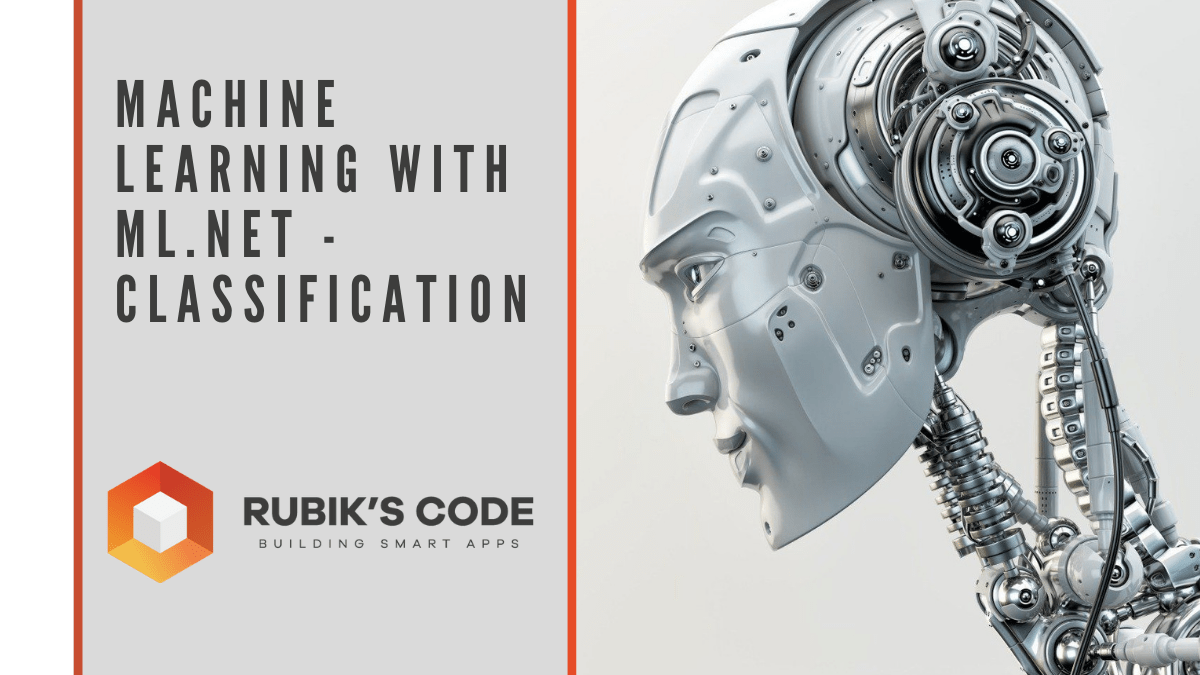## Machine Learning with ML.NET – Ultimate Guide to Classification

In the previous article, we started exploring some of the basic machine learning algorithms and learned how to use ML.NET. There we covered Linear Regression, its variations and we implemented it from scratch with C#. In this article, we focus on the classification...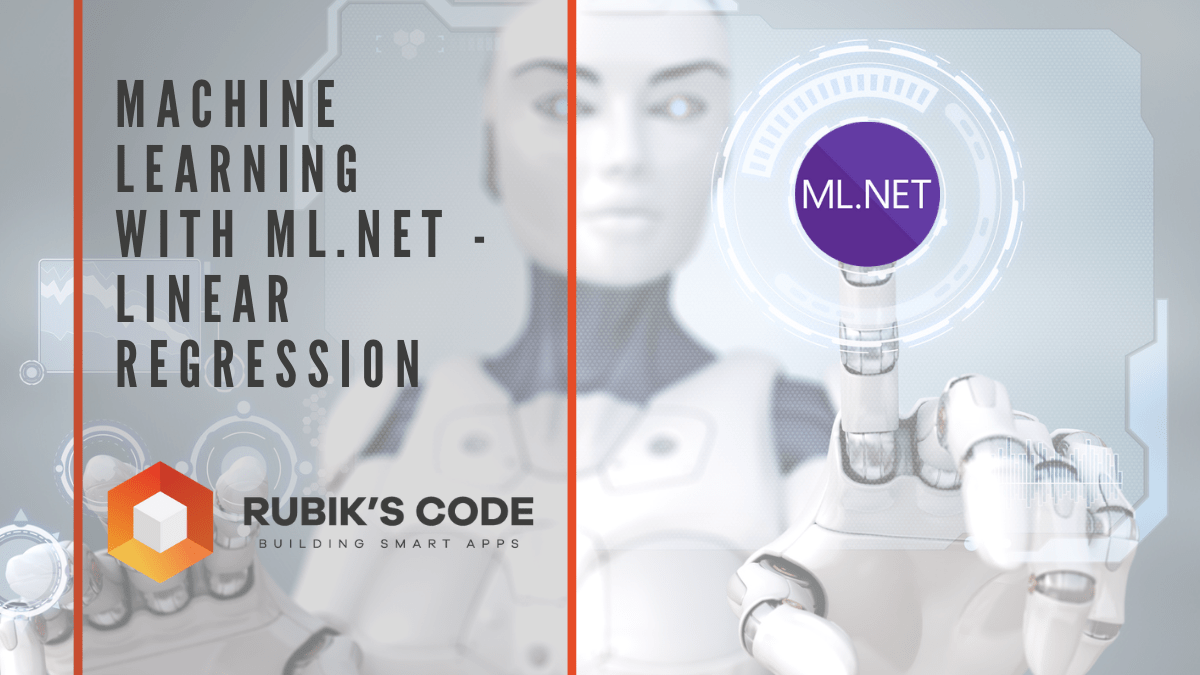## Machine Learning with ML.NET – Linear Regression

Deep Learning and Machine Learning are no longer a novelty. Many applications are utilizing the power of these technologies for cheap predictions, object detection and various other purposes. In this article, we cover the Linear Regression. You will learn how Linear...